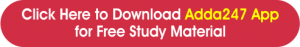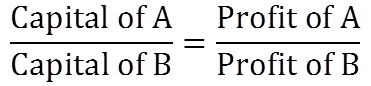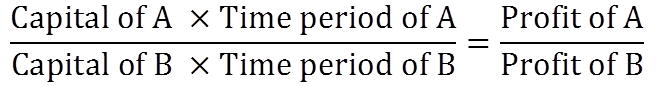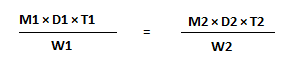Home   »   List of Important Maths Formulas for...

# List of Important Maths Formulas for Defence Exams

## Maths Formulas

In every type of competitive exam, the Mathematics section considers being one of the most important parts to clear the exam and for this practising is the key. Once you know how to solve the question, it becomes a cakewalk for the aspirant and it is the section which can help the candidate in scoring the maximum marks. Whether you are appearing for NDA, CDS, AFCAT, AGNIVEER or any other defence-related exam, Quantitative Aptitude is being judged in almost every exam.

## Maths Formulas for Defence Exams

There can be different forms in which questions are being asked, but these questions are based on the same type of formula and tricks which helps to solve them in the given time constraint. If you are clear with your basics, this section can help you increase your overall score in your specific exam. So to solve these questions knowing the specific formulae for specific questions is the foremost thing. So to help you in the quant section, we are providing you with the important topics of maths and the formulae associated with them. Read the full article to perform better in the exam –## List of Important Mathematics Formulas

Given below are the important topics that you need to cover in order to score good marks in mathematics. We have also provided you with the PDF for every part so that you could practice chapter-wise by applying the required formulas –

### 1. Speed, Distance & Time

This concept has been asked the most in all sorts of competitive exams especially in defence exams and it is also considered to be one of the easiest topics to deal with. however, the type of questions asked in the examinations may have a different variety. But we have provided you with all formulae related to it which will help you to solve every problem related to Speed, distance and time.

1. 1 Kmph = (5/18) m/s
2. 1 m/s = (18/5) Kmph
3. Speed(S) = Distance(d)/Time(t)
4. Average Speed = Total distance/Total Time = (d1+d2)/(t1+t2)
5. When d1 = d2, Average Speed = 2S1S2/(S1+S2), where S1 and S2 are the speeds for covering d1 and d2 respectively.
6. When t1 = t2, Average Speed = (S1+S2)/2, where S1 and S2 are the speeds during t1 and t2 respectively.
7. Relative speed when moving in the opposite direction is S1+S2
8. Relative speed when moving in the same direction is S1-S2
9. A person goes a certain distance (A to B) at a speed of S1 kmph and returns back (B to A) at a speed of S2 kmph. If he takes T hours in all, the distance between A and B is T(S1S2/(S1+S2)
10. When two trains of lengths l1 and l2 respectively travelling at the speeds of S1 & S2 respectively cross each other in time t, then the equation is given as S1+S2 = (l1+l2)/t
11. When a train of length l1 travelling at a speed of S1 overtakes another train of length l2 travelling at speed S2 in time t, then the equation is given as S1-S2 = (l1+l2)/t
12. When a train of length l1 travelling at a speed of S1 crosses a platform/bridge/tunnel of length l2 in time t, then the equation is given as S1 = (l1+l2)/t
13. When a train of lengths l travelling at a speed s crosses a pole/pillar/flag post in time t, then the equation is given as s = l/t
14. If two persons A and B start at the same time from two points P and Q towards each other and after crossing they take T1 and T2 hours in reaching Q and P respectively, then (A’s speed) / (B’s speed) = √T2 / √T1

### 2. Profit & Loss

The profit and Loss formula is used to find out the price of an item and to know how profitable a business is. Every product has a cost price at which an item is purchased and a selling price at which an item is sold and based on the values of these prices, you can easily find out the profit gained or loss incurred on a particular product. Using the formulas given below you can find out the answers to such problems easily –

1. Profit, P = SP – CP; SP>CP
2. Loss, L = CP – SP; CP>SP
3. P% = (P/CP) x 100
4. L% = (L/CP) x 100
5. SP = [(100 + P%)/100] x CP
6. SP = [(100 – L%)/100] x CP
7. CP = [100/(100 + P%)] x SP
8. CP = [100/(100 – L%)] x SP
9. Discount = MP – SP
10. SP = MP -Discount
11. If P% and L% are equal then, %loss = P2/100
12. When the profit is x% and loss is y%, then the net % profit or loss will be: (x-y-xy)/100
13. If a product is sold at x% profit and then again sold at y% profit then the actual cost price of the product will be: CP = [100 x 100 x P/(100+x)(100+y)]. In case of loss, CP = [100 x 100 x P/(100-x)(100-y)]
14. For false weight, profit percentage will be P% = (True weight – false weight/ false weight) x 100
15. When there are two successful profits say x% and y%, then the net percentage profit equals (x+y+xy)/100

### 3. Percentage

Percentage means per hundred. Percentages are a portion or fraction of 100 and it is denoted by the symbol ‘%‘, the percentage is majorly used to compare and find out ratios. The percentage is an interesting and a scoring topic. Once you understand the basics, it becomes easier to solve problems related to percentage –

1. To calculate the percentage of a fraction we have to use the given formula.

Percentage formula = (Numerator/Denominator) ×100

2. To calculate the percentage of a Number.

Percentage /100* Number

3. To express x% as a fraction.

X%/100

To express % as a Fraction, we need to just divide it by 100.

4. To Increase A value or Number by a Given Percentage.

Number * 100+x%/100

5. To Decrease A value or Number by Given Percentage.

Number * 100-x%/100

6. To calculate the percentage Decrement of a number.

Percentage Decreament = Initial value-Final value/ Initial value*100

7. To calculate the percentage increment of a number.

Percentage Increament = Final value – Initial value/ Initial value*100

8. If X% of A is equal to Y% of B.

Z% of A = y*z/x% of B

9. If the passing marks in an examination is P%. If a candidate scores S marks and fails by F marks then Maximum Marks will be.

Maximum Marks = 100*P+S/P

10. If a candidate scores marks and fails by A marks while another candidate scores y% marks and gets B marks more than minimum passing marks, then Maximum Marks will be.

Maximum Marks = Sum of score *100/Difference in % Marks

11.If the price of an item decreases, a person can buy a few Kg more in Y rupees, the actual price of that item.

Actual Price = Rate * Y/100- Rate*X Per Kg

12. If the population of a town is P and it increases at the rate of R% per annum then –Population after ‘n’ years :

Percentage population = P x (1 + R/100)n

13. If the population of a town is P and it decreases at the rate of R% per annum then –Population after ‘n’ years :

Percentage population = P x (1 – R/100)n

14. If the population of a town is P and it increases at the rate of R% per annum then –Population of the town ‘n’ years ago :

Population n years ago = P/(1 + R/100)n

15. If the population of a town is P and it decreases at the rate of R% per annum then –Population of the town ‘n’ years  ago:

Population n years ago = P/(1 – R/100)n

16. Cost of Machine

Value of Machine After n years = P x (1 – R/100)n

Value of Machine Before n years = P/(1 – R/100)n

### 4. Average

Averages can be defined as the central value in a set of data. Average can be calculated simply by dividing the sum of all values in a set by the total number of values. In other words, an average value represents the middle value of a data set. The data set can be of anything like age, money, runs, etc.

1. Average = Sum of quantities/ Number of quantities
2. Sum of quantities = Average * Number of quantities
3. The average of first n natural numbers is (n +1) / 2
4. The average of the squares of first n natural numbers is (n +1)(2n+1 ) / 6
5. The average of cubes of first n natural numbers is n(n+1)2 / 4
6. The average of the first n odd numbers is given by (last odd number +1) / 2
7. The average of first n even numbers is given by (last even number + 2) / 2
8. The average of squares of first n consecutive even numbers is 2(n+1)(2n+1) / 3
9. The average of squares of consecutive even numbers till n is (n+1)(n+2) / 3
10. The average of squares of squares of consecutive odd numbers till n is n(n+2) / 3
11. If the average of n consecutive numbers is m, then the difference between the smallest and the largest number is 2(m-1)
12. If the number of quantities in two groups be n1 and n2 and their average is x and y respectively, the combined average is (n1x+n2y) / (n1+ n2)
13. The average of n quantities is equal to x. When a quantity is removed, the average becomes y.
The value of the removed quantity is n(x-y) + y
14. The average of n quantities is equal to x. When a quantity is added, the average becomes y.
The value of the new quantity is n(y-x) + y

### 5. Algebra

Algebra is a very important section for all competitive exams. It can be a tricky part to solve but using these given formulas you can ace it in this section. Here we are going to mention the list of some important formulas of algebra that will certainly help you to solve Algebra problems

• a² – b² = (a-b)(a+b)
• (a+b)² = a² + 2ab + b²
• (a-b)² = a² – 2ab + b²
• a² + b² = (a-b)² +2ab
• (a+b+c)² = a²+b²+c²+2ab+2ac+2bc
• (a-b-c)² = a²+b²+c²-2ab-2ac+2bc
• a³-b³ = (a-b) (a² + ab + b²)
• a³+b³ = (a+b) (a² – ab + b²)
• (a+b)³ = a³+ 3a²b + 3ab² + b³
• (a-b)³ = a³- 3a²b + 3ab² – b³
• “n” is a natural number, and – bn = (a-b) (an-1 + an-2b +….bn-2a + bn-1)
• “n” is an even number, an + bn = (a+b) (an-1 – an-2b +….+ bn-2a – bn-1)
• “n” is an odd number an + bn = (a-b) (an-1 – an-2b +…. – bn-2a + bn-1)
• (am)(an) = am+n (ab)m = amn

### 6. Partnership

When more than one person invests their money to run a business or firm then this kind of agreement is called partnership. Involved parties in partnership are called partners.

There are two types of partners.

1. Sleeping Partner: Sleeping partner is the person who provides only investment but does not take part in running the business.
2. Working Partner: Working partner is the person who not only invests the money but also takes part in running the business. For this work, he is paid some salary or some percent of profit in addition.
1. If two partners A and B are investing their money to run a business then
(Simple Partnership)Capital of A : Capital of B = Profit of A : Profit of B

2. If two partners A and B are investing their money for different period of time to run a business then
(Compound Partnership)Capital of A × Time period of A : Capital of B × Time period of B = Profit of A : Profit of B

### 7. Time, Work & Wages

When you know Time, Work and wages formula, you can completely link that formula to the solution as soon as you read the question. Knowing Time, Work and wages tricks will also help you solve the questions in a few seconds and thus saving your time for other sections. You can find Time & Work formulas along with important Time & Work Tricks below.

1. Wages are given in proportion to the work done and in indirect (or inverse) proportion to the time taken by the individual.
Total Wage = Total number of days × Wage of 1 day of a person ·
2. Work Done = Time Taken × Rate of Work
3. Rate of Work = 1 / Time Taken
4. Time Taken = 1 / Rate of Work
5. If a piece of work is done in x number of days, then the work done in one day = 1/x
6. Total Work Done = Number of Days × Efficiency
7. Efficiency and Time are inversely proportional to each other
8. X:y is the ratio of the number of men who are required to complete a piece of work, then the ratio of the time taken by them to complete the work will be y:x
9. If x number of people can do W1 work, in D1 days, working T1 hours each day and the number of people can do W2 work, in D2 days, working T2 hours each day, then the relation between them will be### 8. LCM and HCF

LCM i.e. least common multiple is a number which is multiple of two or more than two numbers. For example: The common multiples of 3 and 4 are 12,24 and so on. Therefore, l.c.m.is smallest positive number that is multiple of both. Here, l.c.m. is 12.. HCF i.e. highest common factor are those integral values of number that can divide that number. LCM and HCF problems are very important part of all competitive exams.

Product of two numbers = Their h.c.f. * Their l.c.m.

2) h.c.f. of given numbers always divides their l.c.m.

3) h.c.f. of given fractions =     h.c.f. of numerator
l.c.m. of denominator

4) l.c.m. of given fractions =    l.c.m. of numerator
h.c.f. of denominator

5) If d is the h.c.f. of two positive integer a and b, then there exist unique integer m and n, such that
d = am + bn

6) If p is prime and a,b are any integer then ,This implies   P or P
ab                       a      b

7) h.c.f. of a given number always divides its l.c.m.

## 9. Mensuration 2D and 3D

With the help of the mensuration formulas, you will be able to know and calculate the areas, perimeter, volume, total surface area, curved surface area, length, etc of different geometrical figures. We have provided you with the formulas which are most expected to be used in your specific exam.
Check this out:

 Mensuration Formulas for 2-Dimensional Figures Shape Area Perimeter Circle πr² 2 π r Square (side)² 4 × side Rectangle length × breadth 2 (length + breadth) Scalene Triangle √[s(s−a)(s−b)(s−c), Where, s = (a+b+c)/2 a+b+c (sum of sides) Isosceles Triangle ½ × base × height 2a + b (sum of sides) Equilateral Triangle (√3/4) × (side)² 3 × side Right Angled Triangle ½ × base × hypotenuse A + B + hypotenuse, where the hypotenuse is √A²+B² Parallelogram base × height 2(l+b) Rhombus ½ × diagonal1 × diagonal2 4 × side Trapezium ½ h(sum of parallel sides) a+b+c+d (sum of all sides)

Mensuration Formulas for 3-Dimensional Figures
Shape Area Curved Surface Area (CSA)/
Lateral Surface Area (LSA)
Total Surface Area (TSA)
Cone (1/3) π r² h π r l πr (r + l)
Cube (side)³ 4 (side)² 6 (side)²
Cuboid length × breadth × height 2 height (length + breadth) 2 (lb +bh +hl)
Cylinder π r² h 2π r h 2πrh + 2πr²
Hemisphere (2/3) π r³ 2 π r² 3 π r²
Sphere 4/3πr³ 4πr² 4πr²

### 10. Simple Interest and Compound Interest

Simple interest is based on the principal amount of a loan or deposit. In contrast, compound interest is based on the principal amount and the interest that accumulates on it in every period.

Simple Interest:

1) SI = P x R x T/100

2) Principal = Simple Interest ×100/ R × T

3) Rate of Interest = Simple Interest ×100 / P × T

4) Time = Simple Interest ×100 / P × R

5) If the rate of Simple interest differs from year to year, then

Simple Interest = Principal × (R1+R2+ R3…..)/100

The four variables in the above formula are: SI=Simple Interest P=Principal Amount (This the amount invested)T=Number of yearsR=Rate of interest (per year) in percentage

1. Compound Interest

The difference between the amount and the money borrowed is called the compound interest for a given period of time

1) Let principal =P; time =n years; and rate = r% per annum and let A be the total amount at the end of n years, then

A = P*[1+ (r/100)]n;

CI = {P*[1+ (r/100)]n -1}

2) When compound interest reckoned half-yearly, then r% become r/2% and time n becomes 2n;

= P*[1+ (r/2*100)]2n

3) For the quarterly

A= P*[1+ (r/4*100)]4n

4) The difference between compound interest and simple interest over two years is given by

Pr2/1002or P(r/100)2

5) The difference between compound interest and simple interest over three years is given by

P(r/100)2*{(r/100)+3}

6) When Rates are different for different years, say R1%, R2%, R3% for 1st, 2nd and 3rd year respectively, Then the total amount is given by

P ((1 + R1)/100) ((1 + R2)/100) ((1 + R2)/100)

7) Present worth of Rs. x due n years hence is given by

x/(1+R/100)Thank You, Your details have been submitted we will get back to you.
•Gandhi Jayanti 2022, All You Need to Kno...
•Top 10 Strongest Air Forces of the World
•List of Top 10 Sub Machine Guns In The W...
•Daily Practice Question PDF for Defence ...
•ITBP HC Veterinary Recruitment 2022
•CRPF Recruitment 2022, Notification Out ...